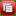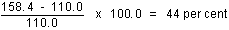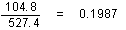6419.0 - Producer and International Trade Price Indexes, 1995
Latest ISSUE Released at 11:30 AM (CANBERRA TIME) 13/02/1995  Ceased
Page tools:Print AllRSSSearch this Product
Contents >> Chapter 16. Dissemination and use of price indexes

Introduction

16.1. This chapter outlines the kinds of price index data available from the ABS and gives some guidance to users who wish to interpret or further manipulate the data.

Publication of statistics

16.2. Producer and international trade price indexes are all compiled and published quarterly. No advance figures are available. In order to ensure that users of these price indexes are treated in a completely even-handed manner, strict security is maintained on the figures before their official publication, and no information about the figures, of any kind, is released before the official embargo time of 11.30 a.m. on the day of publication.

16.3. A large range of data is available via on-line services, diskette, magnetic tape, tape cartridge and CD-ROM. For more details about our electronic data services, contact any ABS Office.

16.4. Price index figures are published for financial years as well as for quarters. The index number for a financial year is the simple arithmetic average (mean) of the index numbers for the 4 quarters of that year. Index numbers for calendar years are not calculated by the ABS but can be derived by calculating the simple arithmetic average of the index numbers for the period concerned.

16.5. All producer and foreign trade price index figures are released as preliminary figures at the time they are first published. Since most of the required basic prices are collected before compilation, the index numbers are not usually subject to revision. However, occasionally revisions may be necessary, as a result of undetected errors in the raw price data, retrospective price changes, or errors in compilation.

16.6. In order to determine actual transaction prices, it is necessary to seek measures of 'special' discounts when they occur, in addition to normal trade discounts. The measurement of special discounts requires continued attention because of the different forms they can take and the variety of ways in which they can be applied. Sometimes these discounts need to be partly estimated and may necessitate revisions to published series.

16.7. As well as various series of index numbers, the ABS also publishes a selection of other figures - percentage changes, index points contributions and index points changes. Each of these measures has already been explained in Chapter 13. Some further explanations to assist users to interpret these figures, or to calculate others for themselves, are given in the following topics.

Percentage changes

16.8. The percentage changes published by the ABS are calculated from the published index numbers. This is because the percentage change is intended merely to summarise the changes in the published index numbers and not as a separate expression of the degree of price change between periods. Thus users who wish to calculate percentage changes for periods not shown in the ABS publications can calculate figures exactly comparable with the ABS figures by deriving them from the published index numbers.

16.9. The percentage change in any price index series for a month is calculated as follows:

% change = ((index (current period) - index (previous period)) / index (previous period) * 100

Similarly, percentage changes between any other pair of periods can be calculated by substituting the periods concerned in the equation.

16.10. Percentage changes across more than one period cannot be calculated by summing the percentage changes for individual periods because this ignores compounding effects. For example:Period Index Number Change from preceding period1 110.02 132.0 + 20 per cent3 158.4 + 20 per centHere the percentage change between period 1 and period 3 is not 40 per cent (20 + 20) but:16.11. Similarly, annual percentage changes cannot be obtained by summing percentage changes for 4 consecutive quarters. Nor can they be obtained by multiplying a single quarter's change by 4. Depending on the precise comparison a user wishes to make, annual changes can be obtained by making either of the following comparisons:
• by calculating the percentage change between the index number for one year (which can be any period of 4 consecutive quarters) and the index number for the preceding year ; or
• by calculating the percentage change between the index number for a particular quarter and the index number for the corresponding quarter of the previous year.

These two calculations, of course, produce different results because they measure different things. The ABS publishes both measures of annual percentage change, i.e. for financial years, and the change from the corresponding month of the previous year.

Index points contributions

16.12. Any detailed analyses of the structure and composition of a price index, or of changes in composition over time, are best done using percentage contributions to the All Groups series, as given in Chapters 4 to 11. However, a common way of looking at changes in composition in the short term, for instance in examining the effects of disparate price movements in different parts of the index, is to look at index points contributions and changes in these contributions, which are produced in the course of compiling the price indexes.

16.13. The index points contribution for any component, in any series, is simply a quantitative expression of how much that component contributes to the magnitude of the index number for the series concerned. For example, when the index number for a particular series stands at 112.5, the size of the contribution of each of its components can be expressed in terms of how many of the 112.5 index points each contributes.

16.14. Most commonly, index points contributions and movements are analysed in terms of the total price index (i.e. in terms of the number of all groups index points contributed by each component to the total index). Index points data are intended to show:

• the relative importance of particular components, classes, groups etc. in the total index at a particular time; and
• the relative contribution of each component, class, group etc. to that period's movement in the total index.
16.15. The contribution of a particular series in a particular period is derived as:
• Weight to total index * index number for the series

Changes in index points contributions are then simply the arithmetic difference between the corresponding contributions in the periods being looked at. For example:Index NumbersPoints ContributionSeries Weight Period 1 Period 2Period 1 Period 2 Period ChangeX 0.3 200 21060 63 + 3Y 0.2 400 39080 78 - 2Z 0.5 300 340150 170 + 20All Groups 1.0 290 311290 311 + 21That is, between periods 1 and 2, the All Groups series increased by 21 index points. Of this movement component Z contributed 20 index points.

Unpublished series

16.16. In addition to the figures contained in the various ABS publications (Catalogue Nos. 6405.0 to 6415.0), a large volume of unpublished figures is available on request. Most of the series included in the weighting pattern for an index are available, subject to confidentiality.

16.17. Special index series that better meet the needs of particular users can also be constructed by the ABS from the detailed unpublished series.

Reference base

16.18. The reference base of each price index is published along with the index series. The reference base for an index is changed as part of the rolling cycle of index rebasing and reweighting.

16.19. For periods following a change in reference base, index numbers on the former basis can still be derived from index numbers on the new basis using arithmetic conversion factors derived from the relationships between the old and new series at the time of the change. For example, from the October 1986 issue onwards, the House Building index was converted to a reference base of 1985-86 = 100.0 from the previous reference base of 1966-67 = 100.0. The factor for Sydney to be used to convert from the new basis to the old was derived as:= 5.0324

16.20. This factor of 5.0324 may be applied to any Sydney House Building index number on the present (1985-86 = 100.0) reference base to give a corresponding index number on the previous (1966-67 = 100.0) reference base. The reverse conversion, i.e. from the previous basis to the present basis, can also be made - in this example using the conversion factor:Use of price index data in contracts

16.21. Sometimes users encounter problems because of the manner in which provisions relating to the use of price indexes are incorporated in contracts or other legal documents, for example, provisions relating to escalation of costs or charges to compensate for price variations. While it is a matter for the parties to decide how such provisions should be worded, and the ABS does not become involved in such decisions, problems could often be avoided if care is taken with respect to a number of specific issues at the time the contract is written. In particular, the following points should be specifically addressed:

• the particular price index series should be clearly specified (i.e. whether it is the series for a particular city, or the weighted average of the six State capital cities, and so on, and whether for the All Groups or a component);
• the index number for the period to be used as the base, or reference point, for the calculation of future changes should be clearly identified; and
• reference should be made to the procedure to be followed in the event of:
• the relevant price index figure being revised by the ABS after it has been used in accordance with the contract provision; or
• the reference base of the published series being changed by the ABS; or
• the specified price index series being discontinued by the ABS.

 Previous Page Next Page